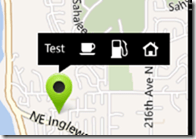# Converting between pixels, meters and map coordinates in Windows Phone

· Igor Kulman

In my current project I needed to solve one quite interesting problem. Imagine you have a map with some pins representing points of interest. If the user taps on a pin, a label with the place title is shown like on this image (that is not from the real project, obviously).The client had an interesting requirement. When the user taps the pin and the label is shown, they wanted the label to be centered on the map (basically move the map so the label appears in the middle of the map).

In Windows Phone you can only center the map to a GeoCoordinate. If you center the map to the tapped pin’s coordinate, it is not quite right, you also need to move it by half the width of the label to the left (and half of the height down). So the solution consists of two steps

1. Convert half of the label width in pixels to meters for a given zoom level (pixels -> meters conversion)
2. Subtract the result from the pin’s coordinate (meters -> degrees conversion)

(and the same for height)

The map component in Windows Phone 8 and 8.1 has quite a nice documentation, so the first step requires some math but is not so difficult.

I created two helper functions to take care of this first conversion

``````public static double MetersToPixels(double meters, double latitude, double zoomLevel)
{
var pixels = meters / (156543.04 * Math.Cos(latitude) / (Math.Pow(2, zoomLevel)));
return Math.Abs(pixels);
}

public static double PixelsToMeters(double pixels, double latitude, double zoomLevel)
{
return pixels  * (156543.04 * Math.Cos(latitude) / (Math.Pow(2, zoomLevel)));
}
``````

The second conversion is more difficult, especially if you want to do it really well. I found a simple solution that works quite ok when you are not right at the poles and used it. The helper function looks like this.

``````public static GeoCoordinate AddMetersToGeoCoordinate(GeoCoordinate current, double metersH, double metersV)
{
return new GeoCoordinate(current.Latitude + (metersH / (111111)), current.Longitude + (metersV / (111111 * Math.Cos(current.Latitude))));
}
``````

Now you just need to put it all together and you are done.